## A 10 kg medicine ball is thrown at a velocity of 15 km/hr ( m/s) to a 50 kg skater who is at rest on the ice. The skater catches

Question

A 10 kg medicine ball is thrown at a velocity of 15 km/hr ( m/s) to a 50 kg skater who is

at rest on the ice. The skater catches the ball and subsequently slides with the ball across the

ice.

in progress 0
5 months 2021-08-10T06:08:15+00:00 1 Answers 159 views 0

## Answers ( )

1. Complete Question

A 10 kg medicine ball is thrown at a velocity of 15 km/hr ( m/s) to a 50 kg skater who is at rest on the ice. The skater catches the ball and subsequently slides with the ball across the  ice.

Calculate the kinetic energy after collision(in joules).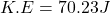Explanation:

From the question we are told that:

Mass of ball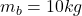Speed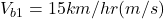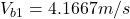Mass of Skater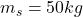Generally the equation for conservation of momentum is mathematically given by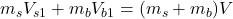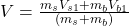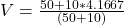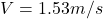Generally the equation for  Kinetic energy is mathematically given by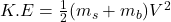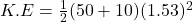Therefore kinetic energy K.E after collision is given as